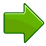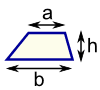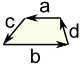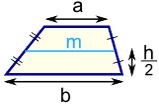# Trapezoid

(Jump to Area of a Trapezoid or Perimeter of a Trapezoid)

A trapezoid is a 4-sided flat shape with straight sides that has a pair of opposite sides parallel (marked with arrows below):Trapezoid Isosceles Trapezoid

A trapezoid:has a pair of parallel sidesis an isosceles trapezoid when it has equal angles from a parallel sideis called a "trapezium" in the UK (see below)

Play with a trapezoid:The parallel sides are the "bases"

The other two sides are the "legs"

The distance (at right angles) from one base to the other is called the "altitude"

## Area of a TrapezoidThe Area is the average of the two base lengths times the altitude: Area = a+b2 × h

### Example: A trapezoid's two bases are 6 m and 4m, and it is 3m high. What is its Area?

Area  =  6 m + 4 m2 × 3 m  =  5 m × 3 m  =  15 m2

The Area of Polygon by Drawing tool is helpful when you can draw your Trapezoid.

## Perimeter of a Trapezoid

The Perimeter is the distance around the edges.The Perimeter is the sum of all side lengths: Perimeter = a+b+c+d

### Example: A trapezoid has side lengths of 5 cm, 12 cm, 4 cm and 15 cm, what is its Perimeter?

Perimeter = 5 cm + 12 cm + 4 cm + 15 cm = 36 cm

## Median of a TrapezoidThe median (also called a midline or midsegment) is a line segment half-way between the two bases. The median's length is the average of the two base lengths: m = a+b2

You can calculate the area when you know the median, it is just the median times the height:

Area = mh

## Trapezium

A trapezium (UK: trapezoid) is a quadrilateral with NO parallel sides.

The US and UK have their definitions swapped over, like this:

 Trapezoid Trapezium US: a pair of parallel sides NO parallel sides UK: NO parallel sides a pair of parallel sides